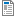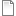## Welcome to the Companion Website

›1. Figure 2.3.docx

›2. Figure 2.3.pdf

›3. Figure 2.4.rtf

›4. Figure 2.4.pdf

›5. Figure 5.1.rtf

›6. Figure 5.1.pdf

›7. Figure 5.2.rtf

›8. Figure 5.2.pdf

›9. Figure 5.4.rtf

›10. Figure 5.4.pdf

›11. Figure 7.1.rtf

›12. Figure 7.1.pdf